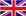English
Blog

### Factors that harm coil inductance

August 06 , 2020
Coil is a more frequently used equipment in power circuits. It is made by winding a transmission line on an insulating tube. The material of the transmission line will endanger the function of the coil. Below I will tell everyone what are the elements that harm coil inductance and what is the function of coil inductance?

What are the elements that harm coil inductance

1. The inductance value in the total control loop with different interface modes is different. When the four coils are connected in parallel, the inductance value is less, and the current in the control loop is larger; when the four coils are connected in series, the control loop inductance is larger. , The control loop current is less. Regardless of the size of the current flowing through each coil or the kinetic energy allocated from the coil, it is better to use the interface method of four coils in reverse series.

2. For coils with iron cores, when two coils are installed at the same iron core, the iron core will cause decision-making hazards to the mutual inductance of the two coils, and because the iron core is a discrete system, its inductance value It is not the only one, the inductance value is different under different current amounts.

What is the function of coil inductance

1. Flow blocking effect

The self-induced electromotive force in the coil inductance has always matched the change in the amount of current in the coil. The coil inductance has a blocking effect on the AC circuit, and the size of the blocking effect is called inductive reactance xl. Its correlation with inductance l and alternating current frequency f is xl=2πfl, and the inductor can be divided into high-frequency choke coil and low-frequency choke coil.

2. Automatic tuning and frequency selection function

Coil inductance and power capacitor in parallel can form an lc tuning power supply circuit. That is, the original oscillating frequency of the power supply circuit is the same as the frequency f of the non-communication AC data signal, and the inductance and capacitive reactance of the control loop are also the same. Therefore, the electromagnetic induction kinetic energy oscillates back and forth between the inductor and the capacitor. This lc control loop is connected in series Resonance condition. In parallel resonance, the inductive reactance and capacitive reactance of the power circuit are equivalent and opposite. The total current in the control loop has less inductive reactance, and the total current flow is larger. The lc coupling circuit has the function of selecting the frequency, which can reduce the frequency of a certain frequency f. The AC data signal is selected.

The function of the inductance coil is blocking current, automatic tuning and frequency selection. The common element that harms coil inductance is the material, and different interface methods cause different inductance.

#### Tags

welcome to ZTC
Talk To ZTC Today To Discuss Your Need For Current Transformers,Get Standard Item, OEM/ODM Service Quote Are Available.